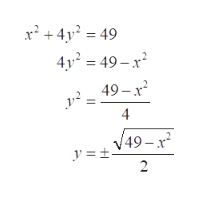# A geometry student wants to draw a rectangle inscribed in the ellipse x2+4y2=49. What is the area of the largest reactangle that the student can draw?

Question
16 views

A geometry student wants to draw a rectangle inscribed in the ellipse x2+4y2=49. What is the area of the largest reactangle that the student can draw?

check_circle

star
star
star
star
star
1 Rating
Step 1

For maximization, equate the derivative of the function to 0 to get the critical points.

Then check the value of function at these critical points. If function is negative then the critical point is the point of maxima. If it is positive, then it is minima otherwise the test fails.

Step 2

For largest area all of the points of the rectangle will touch the ellipse.

Assume any point (x,y) on the ellipse.

Then other horizontal end of one side will be (-x,y) and vertical end of one side will be (x,-y).

So, length of rectangle =2x

Thus, A=(2x)(2y)

=4xy

Step 3

Derive the value of y from th...help_outlineImage Transcriptionclosex' + 4y? = 49 4y² = 49 – x² 49 – x +4) 4 V49-x y =±. 2. fullscreen

### Want to see the full answer?

See Solution

#### Want to see this answer and more?

Solutions are written by subject experts who are available 24/7. Questions are typically answered within 1 hour.*

See Solution
*Response times may vary by subject and question.
Tagged in

### Math